In the financial econometric literature there is significant evidence that stock market returns are, to a certain degree, predictable. The main objective has been to predict the overall level, the conditional mean, of stock returns. However, several studies have shown that only the direction of stock returns is predictable. (Christoffersen 2006)

Prediction of the sign of future returns is known as direction of change forecasting in the literature and is indeed very interesting especially from a trading perspective. The previous literature on direction of change forecasting is mainly based on time series models for excess stock return. For example, Cristoffersen and Diebold 2006 take into account the connection between asset return sign forecast and volatility respectively and show that volatility and higher order conditional moments have statistically significant explanatory power in predicting the sign of future returns. (Quantivity)

Traditionally for this topic probabilities of returns exceeding an upper or lower threshold are estimated, conditioned on an information set based on the previous time period. The majority of the literature has considered univariate return series. However, an alternative approach for estimating these probabilities is to use a multinomial logit model, based on the logisitic function. The basic challenge with the use of this approach is the selection of explanatory variables, since these explanatory variables are part of the information set from the previous time period which helps estimate the probabilities. (Quantivity)

In this paper we use tick-data of an IBM stock from NYSE. A multinomial logit model with penalization is used to predict the direction of change of stock returns. In particular, we take into account varying levels of change by constructing multiple response variables. Response in our models is based on the tick-data and we divide the categories based on a threshold of 1 basis point as following [-2,-1],[-1,0],[0,1],[1,2] and so on. This allows us to not only predict the sign of the return but also particular intervals of returns.

For predicting the probabilities, the use of the multinomial logit model was chosen since it is the most widely used model in the multi-categorical regression. It specifies conditional probabilities of response categories through linear functions of covariate vector X. (Tutz & Zahid 2009) However when the number of predictors is large compared to the observations or when they are highly correlated, the logit model suffers from problems since the estimates of parameters can’t be identified as variance goes to infinity. The use of regularization methods can help to overcome such problems. Regularization methods maximize a penalized log-likelihood. (Tutz & Zahid 2009) Ridge regression, one of the older penalization methods, was defined by Schaefer 1984 for the logistic regression model. Alternative penalization methods have been proposed for univariate GLMs, one of which is Lasso (Tibshirani 1996). In the previous literature for multicategory responses Zhu and Hastie 2004 have used the ridge type penalization and Friedman 2008 uses the L1 penalty (lasso), L2 (ridge) and a mixture of the two (elastic net).

In this paper we make use of two different packages in the R programming language. The MRSP package is used to estimate ridge regression, using fisher scoring, for a multicategory logit model with symmetric-side constraints. While the Glmnet package uses varying levels of alpha ( 0 to 1 ) to estimate Leas Absolute Shrinkage and Selection Operator (LASSO), Ridge estimator and a mixture of two (elastic-net).

Multinomial Logit Model with Symmetric Side Constraints

A multinomial logit model is used when a categorical response variable has more than two categories. Let the response variable Y (1, …, k) have k possible values(categories).

Some additional constraints have to be specified since parameters  are not identifiable. Usually the side constraint is based on a reference category (RSC). In their paper, Tutz and Maqbool use an alternative side constraint that is more appropriate while defining regularization terms i.e. the symmetric side constraint

Parameters for symmetric side constraint are different from the traditional model and therefore have a different interpretation. In this particular case the median response is viewed as the reference category and is defined by the geometric mean.

Hence,  the effects of X on the logits when P (= r|X) is compared to the median response . Especially in high dimensional problems, the use of regularization methods is advantageous because penalized estimators exist and have much better prediction error compared to the usual ML estimator.

GLMNET with LASSO, Ridge and Elastic Net

Friedman et al (2007) introduced fast algorithms for fitting generalized linear models with elastic-net penalties. In our paper we make use of the glmnet package to solve the multinomial regression. The multinomial regression model has already been introduced in the last section. Here we introduce LASSO, Ridge and Elastic Net.Elastic net penalty (Zhu & Hastie 2004) compromises between ridge regression penalty (α = 0) and the lasso penalty (α = 1). This penalty is particularly useful in the p>N situation, or a situation in which there are many correlated predictor variables.

Ridge regression allows coefficients of correlated predictors to borrow strength from each other by shrinking them towards each other. In the unique case of k identical predictors, each gets identical coefficients with 1/kth size that of any single coefficient. (Friedman 2008)

On the other hand, LASSO is indifferent to very correlated predictors and tends to pick one and ignore the rest. In the unique case mentioned above LASSO would break down. The LASSO penalty expects many coefficents to be close to zero and a small number to be larger or non-zero. (Friedman 2008)

The elastic net, a mixture of both, when α = 1 − ε for small ε > 0 performs similar to LASSO, but removes any out-of-the-ordinary behavior caused by extreme correlations. Basically, as α increases from 0 to 1, for a given λ the sparsity of the solution to the minimization problem increases from 0 to the sparsity of the LASSO solution. (Friedman 2008)

DATA AND METHODOLOGY

In our paper we use one month’s tick data of an IBM stock from the NYSE. For the purpose of our analysis we divided the data into two parts, i.e. training data (first 3 weeks) and test data (last one week). We were provided with two data sets, one contained the data on the stock price for each transaction while the other contained the bid and ask price data for every transaction. Both the data sets were consequently merged and the values for which both price and bid-ask data did not exist were ignored. The total number of observations for the bid-ask data set was 287,953. While the total number of observations after merging the two data sets was 74,273. The number of observations for the bid-ask data set is of importance since it was used to create several explanatory variables which helped us in our analysis.

The table on the following page presents all the explanatory variables created for the consequent analysis. A simple explanation about each variable is presented in the table. On average for 4 bid-ask entries there is only one data point for price, therefore, to keep the price data set as big as possible means were taken over four observations.

In our analysis, returns on stock price were categorized based on one-basis-point intervals, i.e. -1 to 0 basis point, 0 to 1 basis point and so on. These intervals were used to create response categories. To allow for robust analysis four different response category variables were constructed, with three, five, seven and nine categories respectively, where each category represents a one-basis-point interval on the return-on-stock price.

As mentioned earlier we made use of two different packages in the R programming language, i.e. MRSP and glmnet. Using MRSP, multinomial logit models with symmetric side constraints and ridge penalization, LASSO and traditional mle were estimated. Therefore, with our four different response category variables a total of twelve models were analyzed with the help of this package. On the other hand, glmnet allows the additional feature of varying the value of alpha from 0 (ridge) to 1 (LASSO) which allowed us to achieve a mix of both penalization (elastic-net) as well. Using 0.1 intervals for alpha a total of eleven different models were estimated. Again with our four response-category variables we were able to obtain 44 different estimates with the glmnet package. With the help of all these different estimates we were able to get predictions on our test data (last week’s data) and obtained Mean Squared Errors for all the models which allowed us to check and compare the errors in all the different predictions to assess accuracy.

MRSP and glmnet use different methods for model selection. In MRSP the model is chosen automatically by Akaike Info Criterion. On the other hand, glmnet uses n (default = 10)fold cross validation to choose an appropriate model. It does so by breaking down data into ten different blocks and uses nine blocks to fit the model and gets the mean squared errors for the rest. Consequently, it chooses the lambda at which the mean squared error is minimized. The cv.function in glmnet allowed us to extract graphs for MSE vs lambda as well. A shortcoming of both the packages was the amount of time they take to run loops, especially for alphas in the case of glmnet. While, an advantage of using both packages is that they do not need a specific reference category but instead use the symmetric multinomial logit model to estimate the models.

It is important to mention here, as shown in the results later, most of the times LASSO tends to leave out majority of the coefficients since it shrinks all the highly correlated coefficients to zero. Most of the coefficients in our analysis are bound to have high correlation since they were all constructed from the same bid-ask data set. On some occasions LASSO shrinks all the coefficients to zero except the intercept which is not of much significance, on the other hand, ridge and elastic net tend to shrink, but not to zero, most of the variables.

Nevertheless, our aim during the entire analysis has been to choose the model with the highest predictive power rather than choosing explanatory variables. This so because our main goal is to approach data as an information set that can predict future return as efficiently as possible. For this reason, we are interested in the model with the lowest mean squared error. Intuitively, as the number of response categories decreases we should expect the MSE to go down but our analysis shows that sometimes such is not the case.The graphs above gives the output from cv.glmnet using the model with nine reference categories. The graph on the left shows coefficient paths for differing values of lambda, i.e. the tuning parameter. Coefficients in this case are in the l-2 norm (the Euclidean norm form). While for the l-1 norm the glmnet gives coefficient vs log lambda graphs for each category. The graph on the right shows the model with ridge penalization using 10-fold cross validation. There are two dotted lines on the graph on the right. The one on the left represents the lambda where the MSE is minimum while the one on the right gives the maximum lambda where one standard error of minimum MSE is still in the range. Our goal in the analysis will be to choose coefficients and model based on the lambda that minimizes the cross validated MSE.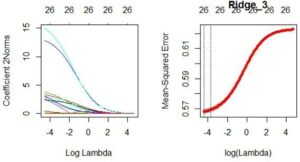The above graphs are for the three category case. The coefficient paths in that case seem similar to the one with the nine category case but the graph on the right side shows a much better fit. The two dotted lines are much closer which is a reflection of a better fit. This in a sense proves our intuition as well that less categories lead to a better fit.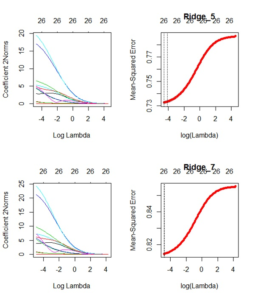The graphs above are for the other two category cases, i.e. five and seven categories respectively. The trend of a better fit with less categories is evident to a certain extent from these graphs as well. However, there is a difference in the distributions of the miniimum MSEs which goes against out early intuition.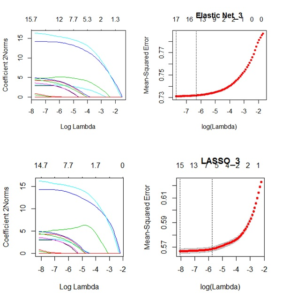The above two graphs are for the model with three response categories. LASSO seems to perform better than the elastic net penalization where alpha = 0.5. LASSO shrinks 11 out of the 27 variables to zero whereas elastic net shrinks 9 variables to zero. While ridge penalization doesn’t shrink any variable to zero, with LASSO and elastic net such is not the case. This goes to show the importance of the right choice of alpha for the analysis. For this very reason we make use of the cv.glmnet function for 11 different values of alpha ranging from 0 to 1 (0.1 intervals) and then choose the model with a better fit and predictive power.

RESULTS and ANALYSIS

As mentioned before we analyze the output of fitted models with the cv.glmnet function. We had four different types of response categories, i.e 3, 5, 7 and 9 response categories.

 9-Category Data Weight of L1 Predicted MSE α = 0.0 2.67258 α = 0.1 2.72469 α = 0.2 2.72583 α = 0.3 2.73232 α = 0.4 2.73291 α = 0.5 2.73329 α = 0.6 2.73248 α = 0.7 2.73383 α = 0.8 2.73194 α = 0.9 3.68399 α = 1.0 2.73746

The table above gives the predicted MSEs for the 9 category data for values of alpha ranging from 0 to 1, where alpha = 0 is ridge penalization and alpha = 1 is LASSO. From the table we can see that the lowest MSE is obtained by using the ridge estimator (alpha=0). From this result we can infer that it is better to keep all 27 variables in the model instead of shrinking some of them to zero. Mean squared errors are obtained by using class (category) predictions.

The set of graphs below provide the cross validation paths for the 9-category model for different values of alpha ranging from 0 to 1. Although the paths seem quite similar but the model with the ridge estimator (first graph) provides a relatively better distribution for the minimum MSE. This means that compared to the other models the value of lambda which minimizes the MSE and the maximum value of lambda within one standard deviation of the minimum MSE are very close. This is indeed a good sign for a better fitted model. Since the MSEs in the graphs are obtained from cross-validation predictions, it only shows the squared prediction errors. Therefore, it is a reasonable idea to check the distribution of the MSEs (in this case the minimum MSE).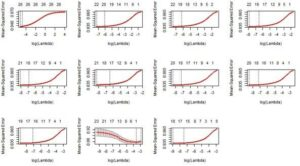To check and compare response variables we now present models with the other three category classification.

 3-Category Data Weight of L1 Predicted MSE α = 0.0 2.77501 α = 0.1 2.78172 α = 0.2 2.78237 α = 0.3 2.78410 α = 0.4 2.78367 α = 0.5 2.78259 α = 0.6 2.78280 α = 0.7 2.78367 α = 0.8 2.78454 α = 0.9 2.78064 α = 1.0 2.78324

The table above provides the predicted MSEs for the model with 3 response categories. Again, using a similar approach, we see that the ridge estimator performs relatively better than the other penalization. It is important to note here that compared to the model with 9 response categories this model gives relatively lower model MSEs but worse predicted MSEs. The set of graphs below present the cross validation paths for the model with 3 response categories for the different values of alpha.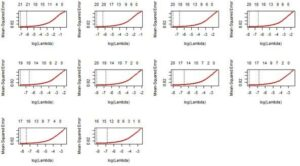Similarly, the table and the set of graphs below provide the predicted MSEs and the cross-validation paths, respectively, for the model with 7 response categories.

 7-Category Data Weight of L1 Predicted MSE α = 0.0 2.68606 α = 0.1 2.72107 α = 0.2 2.72328 α = 0.3 2.72945 α = 0.4 2.73140 α = 0.5 2.72583 α = 0.6 2.72718 α = 0.7 2.72777 α = 0.8 2.72761 α = 0.9 2.72945 α = 1.0 2.73237Similar to the previous two cases, the ridge estimator outperforms the rest in the model with seven response categories as well. Though, in this case it is not clear whether or not the minimum MSE distributions differ between the different values of alpha. As far as predicted mean squared errors go, the model with 7 response categories outperforms the model with 3 response categories but does worse compared to the model with 9 response categories.

The table and set of graphs below provide the predicted MSEs and cross-validation paths, respectively, for our last model with 5 response categories.

 5-Category Data Weight of L1 Predicted MSE α = 0.0 3.00368 α = 0.1 3.00660 α = 0.2 3.00628 α = 0.3 3.00179 α = 0.4 3.00281 α = 0.5 3.00400 α = 0.6 3.00579 α = 0.7 3.00525 α = 0.8 3.00617 α = 0.9 3.00676 α = 1.0 3.00801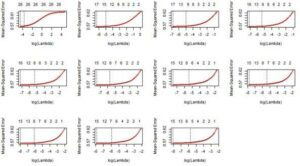The results above show that the predictive power of the model where alpha=0.3 (elastic-net) outperforms the rest. Again there is not much to differentiate between the different plots for the cross-validation paths. Given the lowest value of MSE for alpha=0.3, in this case, it can be inferred that this model is the best with 5 response categories. It should also be noted that compared to the other three models, the model with 5 response categories gives the highest prediction errors.

MRSP

After analyzing the output obtained from the glmnet package we now shift our attention to the other package used during this study, i.e MRSP. MRSP provides the option of estimating a multinomial logit model with symmetric side constraints and ridge penalization. The model provides predicted probabilities for each test observation, with respect to each category. After obtaining these probabilities, expected class is obtained by multiplying these probabilities with the referring class. This allows us to compute the prediction errors, i.e. predicted mean squared errors.

In addition to this we also compute prediction errors for the same model with LASSO and simple multinomial logit (no penalty and reference category = 0). This is done so as to get a comparison with ridge estimator and to understand if any gains to efficiency are obtained. In addition, the tuning parameter, lambda, is chosen using AIC (Akaike Information Criterion) and BIC (Bayesian Information Criterion). The two tables below provide the predicted MSEs obtained using AIC and BIC respectively.

 AIC Category Predicted MSE – LASSO Predicted    MSE   –    No penalty Predicted MSE – Ridge SSC 3 2.52297 2.52256 2.52182 5 2.47749 2.47409 2.47339 7 2.52254 2.51958 2.51893 9 2.80025 2.80066 2.80217

 BIC Category Predicted MSE – LASSO Predicted    MSE   –    No penalty Predicted MSE – Ridge SSC 3 2.52499 2.52256 2.52182 5 2.48389 2.47409 2.47339 7 2.52513 2.51958 2.51893 9 2.79353 2.80066 2.80217

We can infer from the above two tables that the ridge estimator with SSC outperforms the other two models, irrespective of the criterion used. However, for the model with 9 response categories the ridge estimator actually performs the worst. This goes against most of what we found out so far. It should be noted however that for the model with 9 response categories there was an anomaly with glmnet package as well, where the MSE from LASSO was lower than that from the ridge estimator.

CONCLUSION

It can be concluded from the above analysis that the ridge estimator, except for a few cases, outperforms other estimators in achieving the lowest Mean Squared Error. We also found out that our earlier intuition that a smaller number of categories results in a better model was supported by the model MSEs but not by the predicted MSEs.

For our analysis we only had one month of data. We trained 3 weeks of data and tested the last one week. It might have been due to the nature of our data that we obtained certain anomalies which go against the conclusion that the ridge estimator outperforms the rest. However, the majority of the results show that the ridge estimator has the best prediction power and this result is in line with the paper by Tutz and Maqbool (2009). It might be the case that if a similar analysis is performed on a larger data set or at smaller (weekly, daily, intraday) intervals the predictive power improves. For further analysis, increasing the number of categories can be one option but with the limited computational power of the packages an analysis might not be possible. Nevertheless, based on the different techniques used, the multinomial logit model with symmetric side constraints and ridge penalization performed the best based on efficiency of run-time and precision of prediction.

REFERENCES

Christoffersen et al, 2004. Direction-of-Change Forecasts Based on Conditional Variance, Skewness and Kurtosis Dynamics: International Evidence

Friedman, J., Hastie, T., Tibshirani, R., 2008. Regularization paths for generalized linear models via coordinate descent.

Nyquist, H., 1991. Restricted estimation of generalized linear models. Journal of Applied Statistics 40, 133–141.

Schaefer, R., Roi, L., Wolfe, R., 1984. A ridge logistic estimator. Communications in Statistics: Theory and Methods 13, 99–113.

Tibshirani, R., 1996. Regression shrinkage and selection via lasso. Journal of the Royal Statistical Society B 58, 267–288.

Zhu, J., Hastie, T., 2004. Classification of gene microarrays by penalized logistic regression. Biostatistics 5, 427–443.

Direction of Change Forecasting

Tutz, Gerhard, Zahid, M. Faisal, 2009. Ridge Estimation for Multinomial Logit Models with Symmetric Side Constraints. http://www.stat.uni-muenchen.de/

Note: This paper was written with Daniyal Rizwan for Big Data Seminar in Konstanz University

20 May 2020